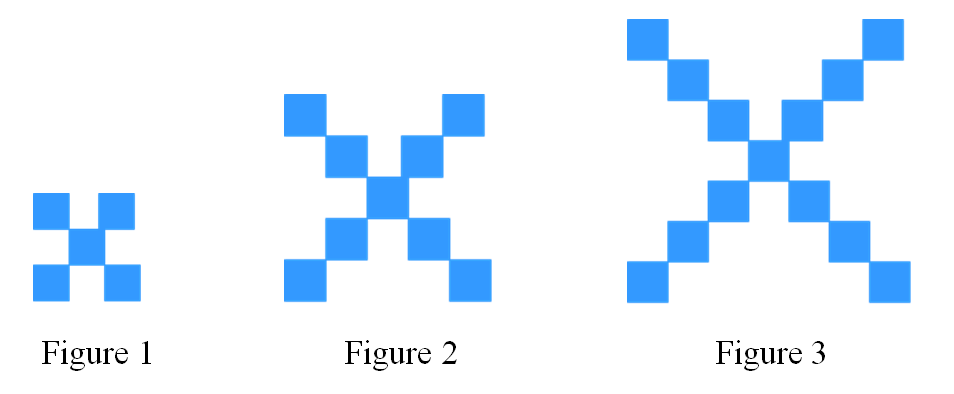# Representing patterns in linear relations

### Representing patterns in linear relations

In this section, we will learn how to represent patterns in linear relations. In other words, these patterns will form a straight line if they are plotted on a graph paper. We can start with creating a table of values which has the independent variable as X, and dependent variable as Y. One way to represent patterns in linear relations is to use the slope-intercept form.

#### Lessons

• 1.a)
Create a table that shows the relationship between the number of squares and the figure number.

b)
Determine the equation that represents the relationship.

c)
Use the equation in b) to find the number of squares in each figure.

d)
How many squares will there be in figure 14?

e)
Determine which figure has 84 squares.

• 2.
Two identical octagons are attached to each other. All the sides in each octagon have the same length.a)
Create a table that shows the number of sides if there are 2,3,4,5 or 6 octagons attached together. Assume each new octagon only attaches to one additional octagon.

b)
Write an equation to calculate the number of sides based on the number of octagons attached together.

c)
How many sides will there be when 15 octagons are attached together?

d)
If there are 84 sides in total, how many octagons are attached together?

• 3.
Write the linear equation that represents the relationship of each set of numbers.
a)
 x y 1 16 2 20 3 24 4 28

b)
 x y 1 -2.1 2 0.7 3 3.5 4 6.3

c)
 x y 0 9 1 18 2 27 3 36

d)
 x y 0 -1.4 1 -4.6 2 -7.8 3 -11